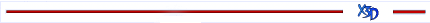5.1, Table 5.1

Replace Table 5.1 with the following:

5.8

Insert the following new field types after 5.8 and renumber the remaining subclauses:

#5.9 SFMatrix3d and MFMatrix3d

The SFMatrix3d field specifies a 3×3 matrix of double-precision floating point numbers. MFMatrix3d specifies zero or more 3×3 matrices of double-precision floating point numbers. Each floating point number is represented in the X3D file as specified in the respective encoding.

SFMatrix3d matrices are organized in row-major fashion. The first row of the matrix stores information for the x dimension, and the second for the y dimension. Since these data types are commonly used for transformation matrices, translation values are stored in the third row.

The initial value of an uninitialized SFMatrix3d field is the identity matrix [1 0 0 0 1 0 0 0 1]. The initial value of an uninitialized MFMatrix3d field is the empty list.#5.10 SFMatrix3f and MFMatrix3f

The SFMatrix3f field specifies a 3×3 matrix of single-precision floating point numbers. MFMatrix3f specifies zero or more 3×3 matrices of single-precision floating point numbers. Each floating point number is represented in the X3D file as specified in the respective encoding.

SFMatrix3f matrices are organized in row-major fashion. The first row of the matrix stores information for the x dimension, and the second for the y dimension. Since these data types are commonly used for transformation matrices, translation values are stored in the third row.

The initial value of an uninitialized SFMatrix3f field is the identity matrix [1 0 0 0 1 0 0 0 1]. The initial value of an uninitialized MFMatrix3f field is the empty list.#5.11 SFMatrix4d and MFMatrix4d

The SFMatrix4d field specifies a 4×4 matrix of double-precision floating point numbers. MFMatrix4d specifies zero or more 4×4 matrices of double-precision floating point numbers. Each floating point number is represented in the X3D file as specified in the respective encoding.

SFMatrix4d matrices are organized in row-major fashion. The first row of the matrix stores information for the x dimension, the second for the y dimension, and the third for the z dimension. Since these data types are commonly used for transformation matrices, translation values are stored in the fourth row.

The initial value of an uninitialized SFMatrix4d field is the identity matrix [1 0 0 0 0 1 0 0 0 0 1 0 0 0 0 1]. The initial value of an uninitialized MFMatrix4d field is the empty list.#5.12 SFMatrix4f and MFMatrix4f

The SFMatrix4f field specifies a 4x4 matrix of single-precision floating point numbers. MFMatrix4f specifies zero or more 4x4 matrices of single-precision floating point numbers. Each floating point number is represented in the X3D file as specified in the respective encoding.

SFMatrix4f matrices are organized in row-major fashion. The first row of the matrix stores information for the x dimension, the second for the y dimension, and the third for the z dimension. Since these data types are commonly used for transformation matrices, translation values are stored in the fourth row.

The initial value of an uninitialized SFMatrix4f field is the identity matrix [1 0 0 0 0 1 0 0 0 0 1 0 0 0 0 1]. The initial value of an uninitialized MFMatrix4f field is the empty list.”

5.16

In the third sentence of paragraph 1, replace "pair" with "3-tuple".

5.16

Insert the following new field types after 5.16:

#5.21 SFVec4d and MFVec4d

The SFVec4d field or event specifies a three-dimensional (3D) homogeneous vector. An MFVec4d field or event specifies zero or more 3D homogeneous vectors. SFVec4d's and MFVec4d's are represented as a 4-tuple of double-precision floating point values (see 5.5 SFDouble and MFDouble). The allowable form for a double-precision floating point number is defined in the specific encoding.

The initial value of an uninitialized SFVec4d field is (0 0 0 1). The initial value of an MFVec4d field is the empty list.#5.22 SFVec4f and MFVec4f

The SFVec4f field or event specifies a three-dimensional (3D) homogeneous vector. An MFVec4f field or event specifies zero or more 3D homogeneous vectors. SFVec4f's and MFVec4f's are represented as a pair of single-precision floating point values. The allowable form for a single-precision floating point number is defined in the specific encoding.

The initial value of an uninitialized SFVec4f field is (0 0 0 1). The initial value of an MFVec4f field is the empty list.”